# Sanscript

Implementation of programming language Sanscript

Sanscript is the native (and the only) IDE for Sansript programming language. Developed by Northwoods Software, it was abandoned and made free in April 2000. No further support is provided. Sanscript is written in C++/MFC.

## Examples:

Example for versions Sanscript 2.2

Sanscript is a fully visual programming language, so no source code is available. See screeshots instead.

This example contains a lot of branches on condition, and in Sanscript each branch is described in a new flowgram.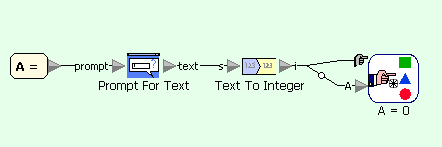Quadratic equation example in Sanscript (main flowgram)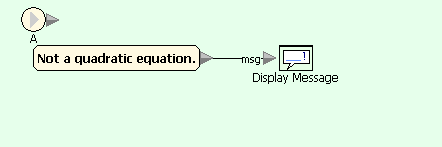Quadratic equation example in Sanscript (on condition A=0)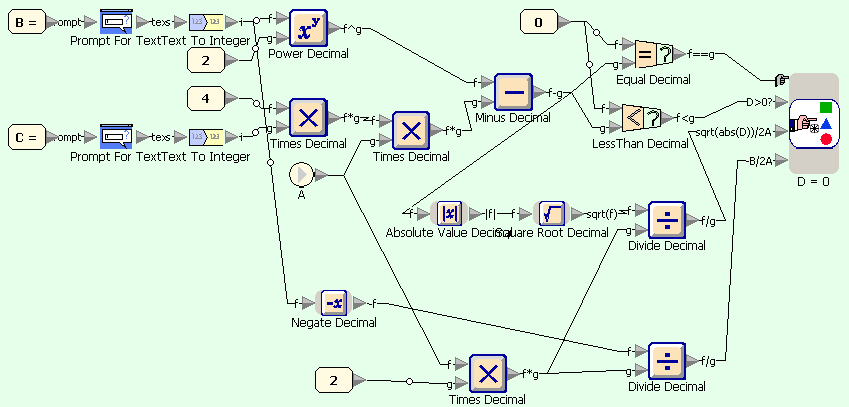Quadratic equation example in Sanscript (on condition A=default)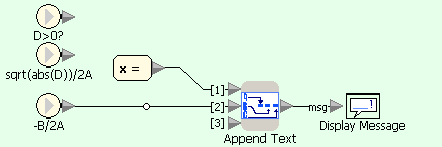Quadratic equation example in Sanscript (on condition D=0)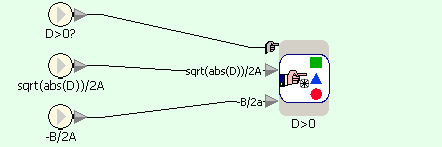Quadratic equation example in Sanscript (on condition D=default)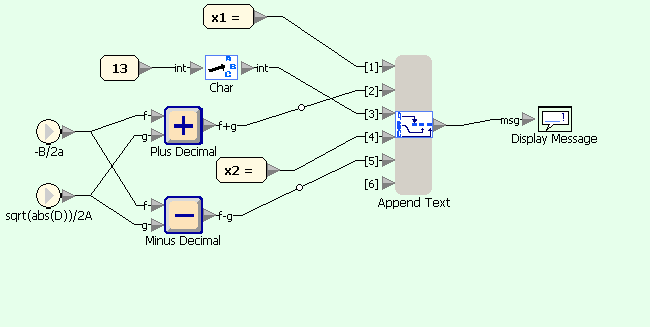Quadratic equation example in Sanscript (on condition D>0)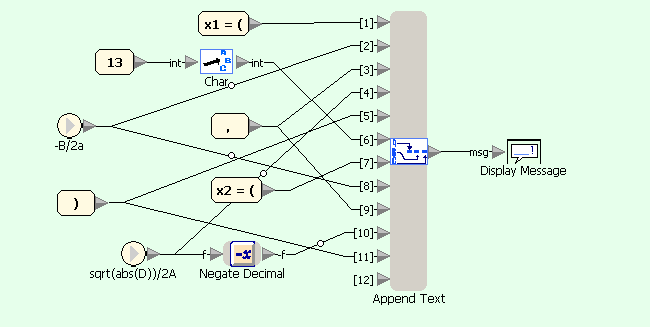Quadratic equation example in Sanscript (on condition D<0)

### Fibonacci numbers:

Example for versions Sanscript 2.2

Sanscript is a fully visual programming language, so no source code is available. See screeshots instead.

Fibonacci numbers are calculated in the same way as factorial: a loop calculates a list of numbers, starting with two first ones, and then this list is concatenated to produce the output. Within the loop current number is added to the list and replaced with next number, while next number is replaced with a sum of current and next numbers.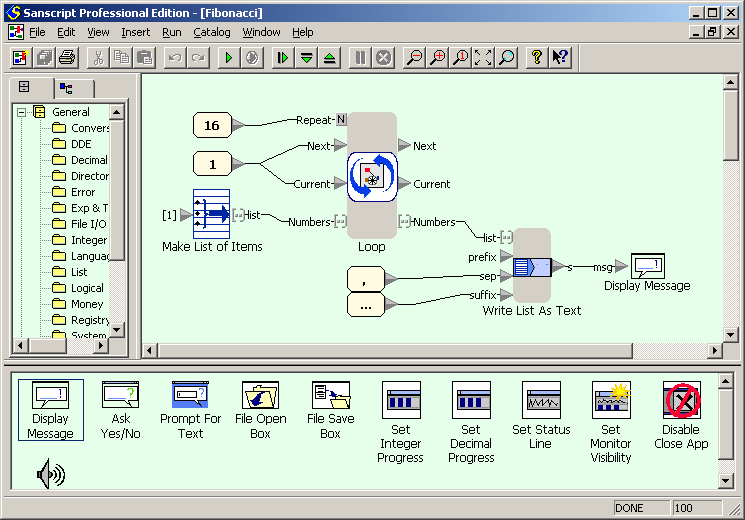Fibonacci numbers example in Sanscript (flowgram)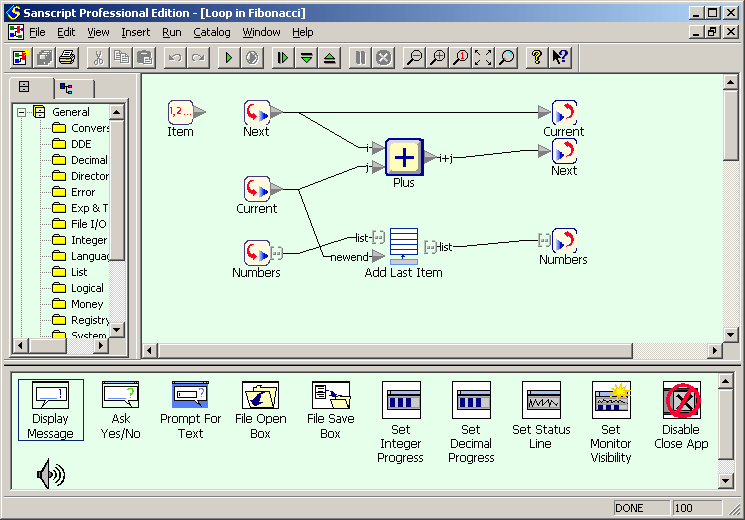Fibonacci numbers example in Sanscript (repeat)

### Factorial:

Example for versions Sanscript 2.2

Sanscript is a fully visual programming language, so no source code is available. See screeshots instead.

First screenshot shows the flowgram of the example. The main part of it is “Repeat” function (an analogue of loop in other languages) which calculates the factorials of numbers from 1 to 16 and stores them in a list. This function has two inlet/outlet pairs which correspond to global variables in other languages: `factorial` and `message`. `factorial` is an integer which stores the current value of factorial. `message` is a list of text lines `n! = (n!)`. The “Repeat” function has one more inlet `N` which defines the number of iterations to be done. The inlets are initialized with constants and an empty list, and the loop starts. Once the calculations are done, `message` has to be displayed. This can be done using `Write List as Text` function, which writes all elements of list into a single text variable. The separator between elements of the list is defined with `sep` inlet; in this case it is newline character, generated using “Char” function which converts an ASCII-code into a corresponding character. Finally, the concatenated list is displayed using “Display Message” function.

Second screenshot shows the intrinsics of “Repeat” function. Element marked with “1, 2 …” is loop counter, started with 1 and incremented by 1 each iteration. On each iteration `factorial` is multiplied by the value of loop counter (using “Times” function). After this its new value is concatenated with loop counter and “! = ” constant string (using “Append Text” function) to produce one line of output. Finally, this line is added to the list `message` (using “Add Last Item”) function.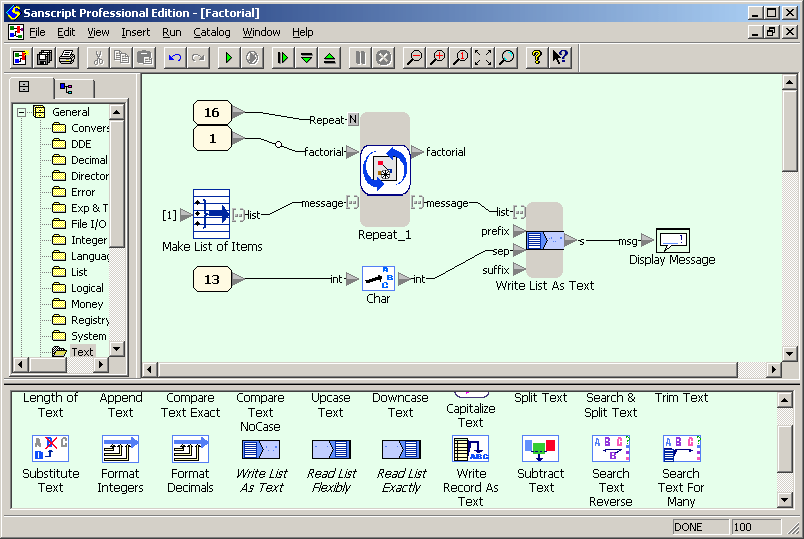Factorial example in Sanscript (flowgram)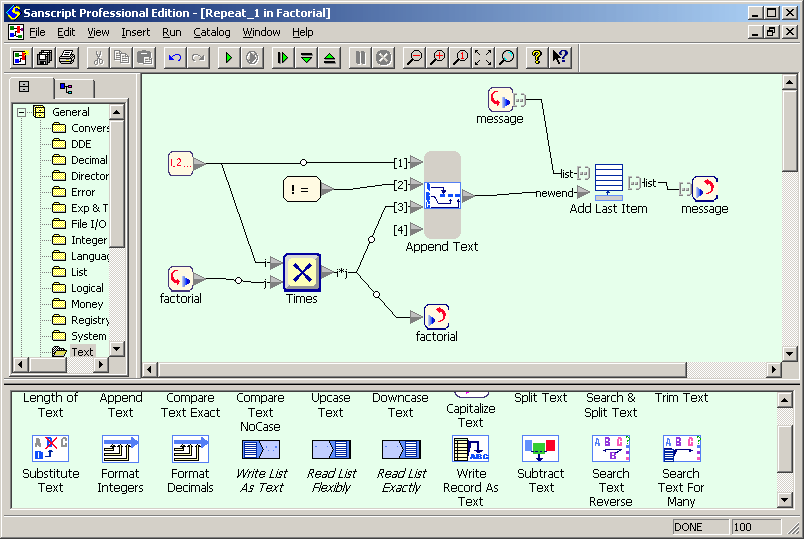Factorial example in Sanscript (repeat)

### Hello, World!:

Example for versions Sanscript 2.2

Sanscript is a fully visual programming language, so no source code is available. See screenshot instead.

The flowgram (Sanscript equivalent of program) for this example consists of two functions: constant “Hello, World!” and function “Display Message”. Once the flowgram runs, the constant function produces the message on its outlet. An arrow link connects this outlet to inlet of “Display Message” function, so it creates a pop-up window with this message.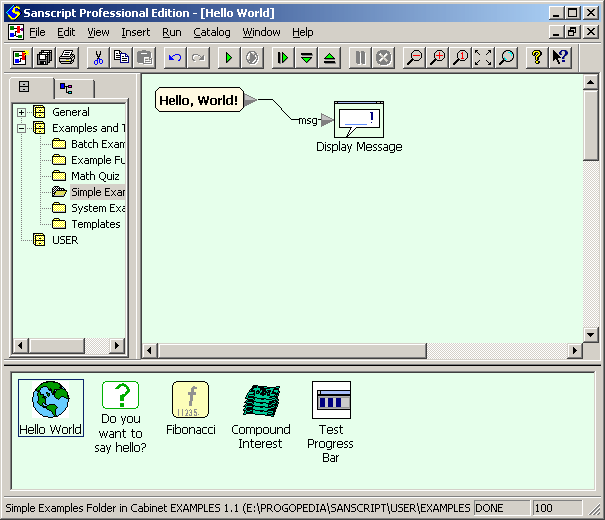"Hello, World!" example in Sanscript

### CamelCase:

Example for versions Sanscript 2.2

First flowgram converts user-entered text in lowercase and passes it into a character-wise processing loop. Second diagram uses `Split Text` block for extracting next character of the string, which is then compared with characters with ASCII-codes before a and after z, and the result of comparison is passed to selection block. Third diagram shows processing of letters: depending on the value of `lastSpace` either the letter or its uppercase equivalent are appended to the result, and `lastSpace` is set to `FALSE`. Fourth diagram shows processing of non-letter characters: it only sets the value of `lastSpace` to `TRUE`.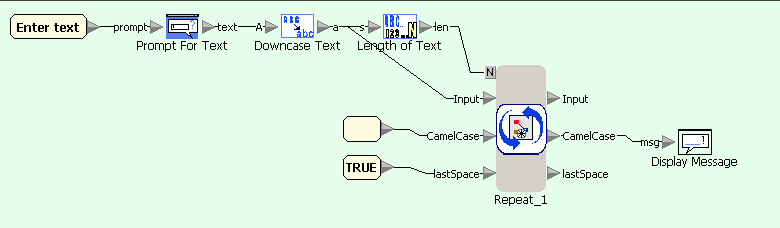CamelCase example in Sanscript (main flowgram)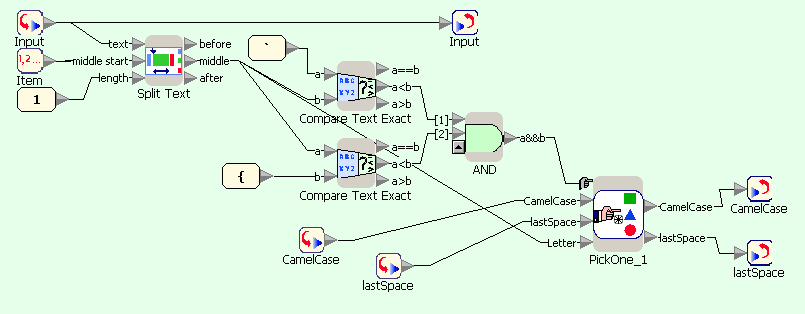CamelCase example in Sanscript (repeat block)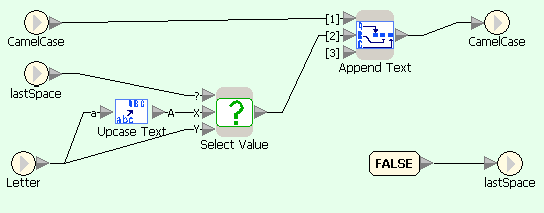CamelCase example in Sanscript (if character is letter)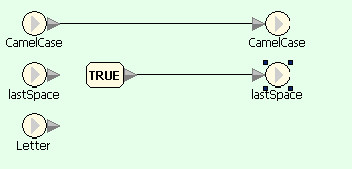CamelCase example in Sanscript (if character is not a letter)